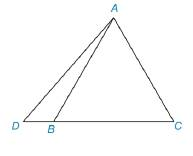Chapter 3.5, Problem 26E### Elementary Geometry for College St...

6th Edition
Daniel C. Alexander + 1 other
ISBN: 9781285195698

#### Solutions

Chapter
Section### Elementary Geometry for College St...

6th Edition
Daniel C. Alexander + 1 other
ISBN: 9781285195698
Textbook Problem
1 views

# In Exercises 25 and 26, complete each proof shown on page 160. Given: Equilateral Δ A B C and D - B - C Prove: D A > A CPROOF Statements Reasons 1. ? 1. Given 2. Δ A B C is equiangular, so m ∠ A B C = m ∠ C 2. ? 3. m ∠ A B C > m ∠ D ( ∠ D of Δ A B D ) 3. The measure of an exterior ∠ of a Δ is greater than the measure of either nonadjacent interior ∠ 4. ? 4. Substitution 5. ? 5. ?

To determine

To prove:

The side DA is greater than the side AC.

Explanation

Given:

Consider the figure,

Figure (1)

From figure (1),

Equilateral ΔABC and D-B-C

If the measure of one angle of a triangle is greater than the measure of a second angle, than the side opposite to the larger angle is longer than the side opposite to the smaller angle.

The measure of an exterior angle of a triangle is greater than the measure of either nonadjacent interior angle of a triangle.

Definition:

The triangle whose all the sides are equal and the measures of all the angle are same, that triangle is called equilateral or equiangular triangle.

Approach:

Consider an equilateral ΔABC,

Since, in equilateral triangle the measures of all the angle are same, then

mABC=mC(1)

Consider the triangle ΔABD,

Since, the measure of an exterior angle of a triangle is greater than the measure of either nonadjacent interior angle of a triangle. Then,

mABC>mD(2)

Substitute equation (1) in equation (2).

mC>mD(3)

Since, the measure of one angle of a triangle is greater than the measure of a second angle, than the side opposite to the larger angle is longer than the side opposite to the smaller angle. Then, from equation (3) the side DA is greater than the side AC. So,

DA>AC

 PROOF Statements Reasons 1. Equilateral ΔABC and D-B-C 1

### Still sussing out bartleby?

Check out a sample textbook solution.

See a sample solution

#### The Solution to Your Study Problems

Bartleby provides explanations to thousands of textbook problems written by our experts, many with advanced degrees!

Get Started

#### The simplified form of the derivative of y=(x+11−x2)3.

Mathematical Applications for the Management, Life, and Social Sciences

#### In Exercises 1520, simplify the expression. 19. (3xy24x3y)2(3xy32x2)3

Applied Calculus for the Managerial, Life, and Social Sciences: A Brief Approach

#### True or False: If converges absolutely, then it converges conditionally.

Study Guide for Stewart's Single Variable Calculus: Early Transcendentals, 8th